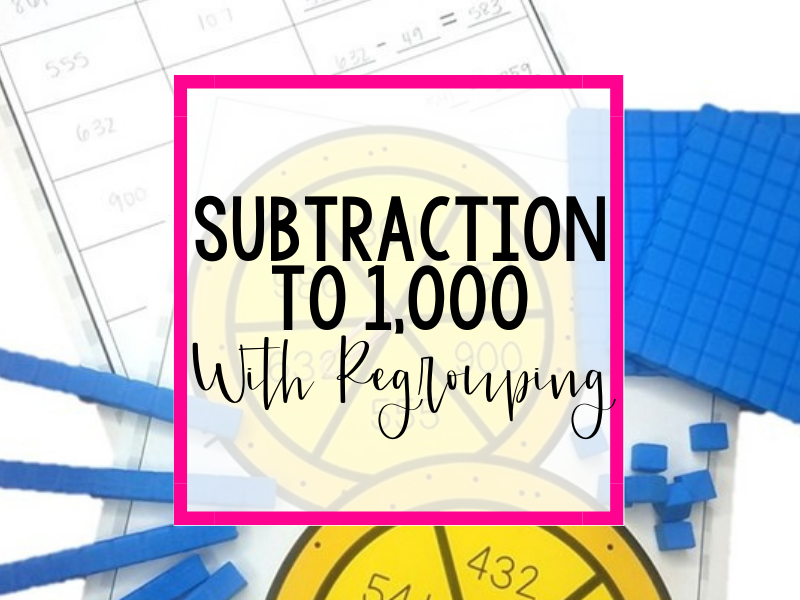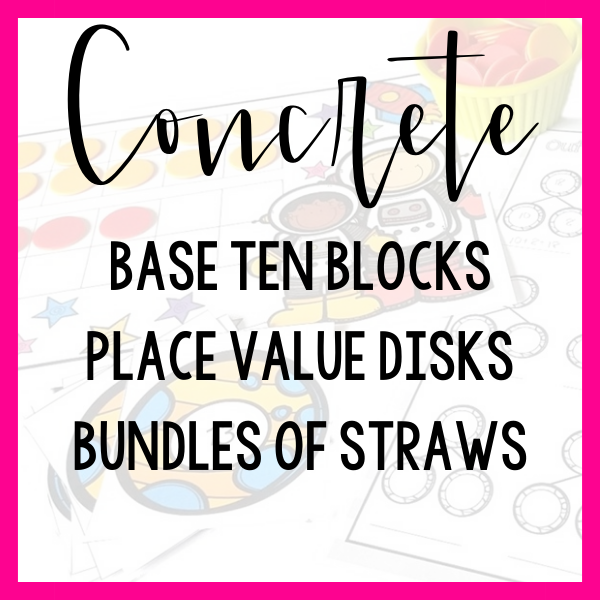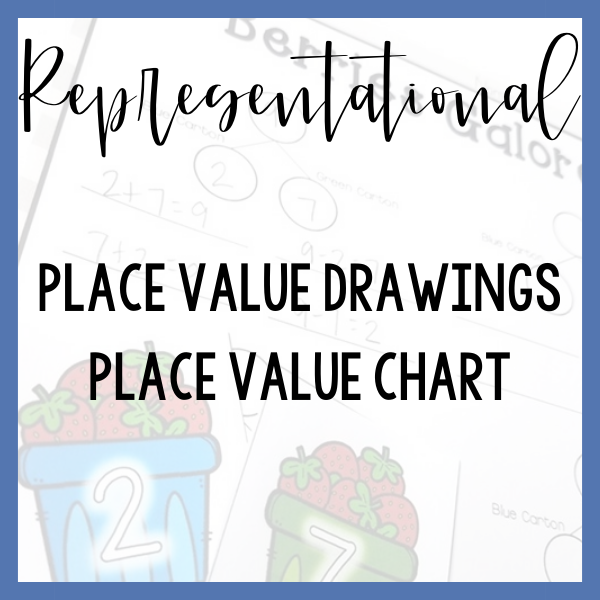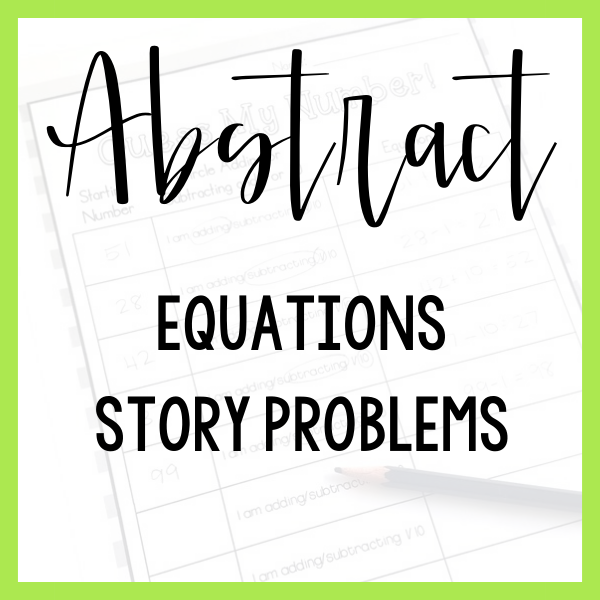# subtraction to 1,000 with regrouping

It is important to note that the 2nd-grade standard does NOT ask that students master the written standard algorithm for adding and subtracting numbers to 1,000! In fact, students are only required to relate the strategy to a written methodThe 5 examples listed are certainly not an exhaustive list of tools and lessons that could be used to teach this skill.

They are rather a sample progression from hands-on to abstract thinking!

Which step represents your students’ current level of understanding?

Base ten blocks are a proportional model. This means that as your students are working with the material their thinking is supported with visual feedback. When they change ten ones in for a ten the size of the material looks (and is!) correct.

**When you are subtracting numbers to 100 I recommend that you start with a groupable model such as linking cubes. When using base ten blocks to subtract to 1,000 your students won’t physically be able to decompose a hundred into tens or a ten into ones- base ten blocks are “pre-grouped”. If you think your students need more time with a groupable model, consider spending more time in subtraction to 100 before moving to 3-digit subtraction.

Support your students futher by asking them to model subtraction with base ten blocks on a place value chart.

Because you have the goal of ultimately relating this place value strategy to the written algorithm, you will want your students to organize their base ten blocks on a place value chart in the same vertical configuration that they will ultimately use when following the standard algorithm for subtraction.

Frequently ask your students to relate their model to the meaning of subtraction – How is this model helping us to take away, take apart or find the difference?

You will want to support your students in moving from a proportional model (base ten blocks) to a non-proportional model such as  place value disks.

In moving toward a non-proportional model, you are still supporting your students with a concrete, hands-on experience but you are lessening the support provided by the manipulative in order to move your students towards abstract thinking.

Think of this as taking baby steps away from hands-on materials.

When using a pre-grouped model, continue to arrange your materials on a place value chart to mimick the ultimate organization of the standard algorithm.

Introduce the standard algorithm directly alongside hands-on materials that your students have been successful in using.

As your students model with hands-on materials, think aloud and reflect back the actions they have taken, one step at a time, and record their thinking using the standard algorithm.

For example: You took 5 ones away from the 9 ones in 239 and found that you had 4 ones left. Over here, I will record 4 ones because 9 ones – 5 ones = 4 ones.

As you complete problems, ask questions of your students that require them to link their understanding of hands-on materials with the written method.

• I see you built 239 using place value disks. Where do we see the 239 in our equation?
• I see you subtracted 1 ten from 3 tens using your blocks. Where did we show that in our equation?

While your students are now able to relate a hands-on method to a written method, you want to take a step back and move your students from hands-on supports to representative models.

In this case, a place value drawing on a place value chart can be a supportive model for your students.

Begin by asking your students to model an addition equation using place value disks. As they are working, record their actions, one step at a time, as a place value drawing on a place value chart.

The process and questioning is very similar to the process for linking hands-on and written methods.

Although your students have now had exposure to subtraction with regrouping using hands-on materials, place value drawings and the written algorithm, you have not explicitly linked the representative drawing with the written algorithm- don’t skip this step!

Think of your students understanding of math concepts as a large web. Each strategy, method and skill is it’s own point on the web. The web is strengthened when these points are linked together.

Just as you used hands-on materials alongside the written method, repeat this process by asking your students to solve using a place value drawing alongside the written method.

Again, focus on asking linking questions that require students to articulate how they put two numbers together using each of these methods.

# Tools To USe